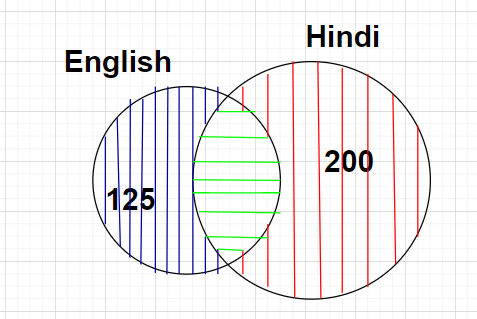Courses
Courses for Kids
Free study material
Free LIVE classes
MoreLIVE
Join Vedantu’s FREE Mastercalss

# In a group of 500 people, 200 can speak Hindi alone while only 125 speak English alone. The number of people who can speak both Hindi and English is${\text{A}}{\text{. 175}} \\ {\text{B}}{\text{. 325}} \\ {\text{C}}{\text{. 300}} \\ {\text{D}}{\text{. 375}} \\$Verified
361.2k+ views
Hint: Here, we will proceed by drawing the relevant Venn diagram according to the problem statement and then finding out the value of the unknown regions by visually analysing the diagram.Given, there are total 500 number of people in the group who can speak either English or Hindi or both Hindi and English (this region is represented by all the three coloured lines which are blue, green and red lines in the figure or the complete region of the figure) i.e., $n\left( {E \cup H} \right) = 500$
Also given that 200 number of people can speak Hindi alone (this region is represented by red coloured lines in the figure) i.e., $n\left( {H{\text{ only}}} \right) = 200$
Also given that 125 number of people can speak English alone (this region is represented by blue coloured lines in the figure) i.e., $n\left( {E{\text{ only}}} \right) = 125$
We have to find the number of people who can speak both Hindi and English (this region is represented by green coloured lines in the figure) i.e., $n\left( {E \cap H} \right)$
Clearly from the Venn diagram shown, we can write
Total region which consists of all the coloured lines which includes blue, green and red coloured lines is equal to the sum of the region which consists of blue coloured lines, the region which consists of green coloured lines and the region which consists of red coloured lines.
$\Rightarrow n\left( {E \cup H} \right) = n\left( {E{\text{ only}}} \right) + n\left( {E \cap H} \right) + n\left( {H{\text{ only}}} \right) \\ \Rightarrow n\left( {E \cap H} \right) = n\left( {E \cup H} \right) - n\left( {E{\text{ only}}} \right) - n\left( {H{\text{ only}}} \right) = 500 - 125 - 200 \\ \Rightarrow n\left( {E \cap H} \right) = 175 \\$
Therefore, the total number of people who can speak both Hindi and English are 175
Hence, option A is correct.

Note: In this particular problem, the total number of people who can speak Hindi (this region is represented by two coloured lines which are red and green) is $n\left( H \right) = 200 + 175 = 375$ and the total number of people who can speak English (this region is represented by two coloured lines which are blue and green) is $n\left( E \right) = 125 + 175 = 300$.
Last updated date: 24th Sep 2023
Total views: 361.2k
Views today: 10.61k